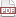Tip 82: Insert NA, NaN, and other markers into your data set

14 March 12. [link]PDF version

level: somewhat advanced data trickster

purpose: leave notes

Part of a series of tips on POSIX and C. Start from the tip intro page, or get 21st Century C, the book based on this series.

The IEEE floating-point standard, which is how your computer represents numbers, has a form for NaN, which indicates a math error, like $0/0$ or $\ln(-3)$.

In fact, it has a lot of forms for NaN: the sign bit can be zero or one, then the exponent is all ones, and the rest is nonzero, so you have a bunch of bits like this: S11111111MMMMMMMMMMMMMMMMMMMMMMM, where S is the sign and M the unspecified mantissa.

That gives us a whole lot of room to play around, because we can specify those Ms to be anything we want (as long as it's nonzero, because zero mantissa indicates $\pm$ infinity, depending on the sign bit). Once we have a way to control those free bits, we can add all kinds of distinct semaphores into a cell in a numeric grid.

The little program below generates and uses an NA marker. The trick is primarily in set_na, so focus your attention there first. We first use the nan function to generate a NaN with a specific bit pattern matching the input string.

Now we have a bit pattern that is a NaN, but a very specific one, and one that the system didn't generate. Now the is_na function just needs to check whether the bit pattern of the number we're testing matches the special bit pattern that we made up. We do this by treating both inputs as character strings and doing character-by-character comparison.

/* Compile with:
export CFLAGS="-g -Wall -std=gnu11 -O3"  #the usual.
make na
*/
#include <stdio.h>
#include <math.h> //isnan

double ref;

double set_na(){
if (!ref) ref=nan("21");
return ref;
}

int is_na(double in){
if (!ref) return 0;  //set_na was never called==>no NAs yet.

char *cc = (char *)(&in);
char *cr = (char *)(&ref);
for (int i=0; i< sizeof(double); i++)
if (cc[i] != cr[i]) return 0;
return 1;
}

int main(){
double x = set_na();
double y = x;
printf("Is x=set_na() NA? %i\n", is_na(x));
printf("Is x=set_na() NAN? %i\n", isnan(x));
printf("Is y=x NA? %i\n", is_na(y));
printf("Is 0/0 NA? %i\n", is_na(0/0.));
printf("Is 8 NA? %i\n", is_na(8));
}
discussion

My NA marker is a type of NaN; not all NaNs are NAs. This seems logical and correct to me: if the data is missing, we can't do math on it; a math error is from mis-processing extant data, not a missing data point. The R system does it the other way `round: NaN is a type of NA, but NA is not a type of NaN. They're smart people who have good reasons for (most of) what they do, but this seems backward to me. I'm not sure how I'd implement their version using the tool here.

I produced a single semaphore to store in a numeric data point, using "21" as the key element of the marker. Given that there are several other strings besides 21, we can insert as many distinct markers directly into our data set as we please. SAS users love to do this, inserting .a through .zs in their data sets for all sorts of exceptions.

I've inquired to many geeks about whether they actually use the distinction between NaN and NA, like whether they write code of the form if (is.NA) do this; else if(is.NAN) do something else, and I couldn't find a single person who does. And so, a question for you, the reader: given the ability to place arbitrary semaphores in a matrix of numbers, what would you use it for?